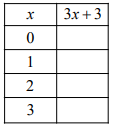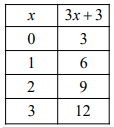mycollegehive
Complete the table for the given values of x.
JMAP math expression0Complete the table for the given values of x.29 viewsShareFollowUniversity of Benin Nigeria
25 June 2020University of Lagos Nigeria
12 August 202003x + 3

When x = 0, the expression becomes:

3(0) + 3

= 3

when x = 1,

3(1) + 3

= 3 + 3

= 6

when x = 2,

3(2) + 3

= 6 + 3

= 9

when x = 3,

3(3) + 3

= 9 + 3

= 12Share### Related Tags

JMAP

2 followers

141 questionsmath

2 followers

990 questionsexpression

1 followers

36 questions